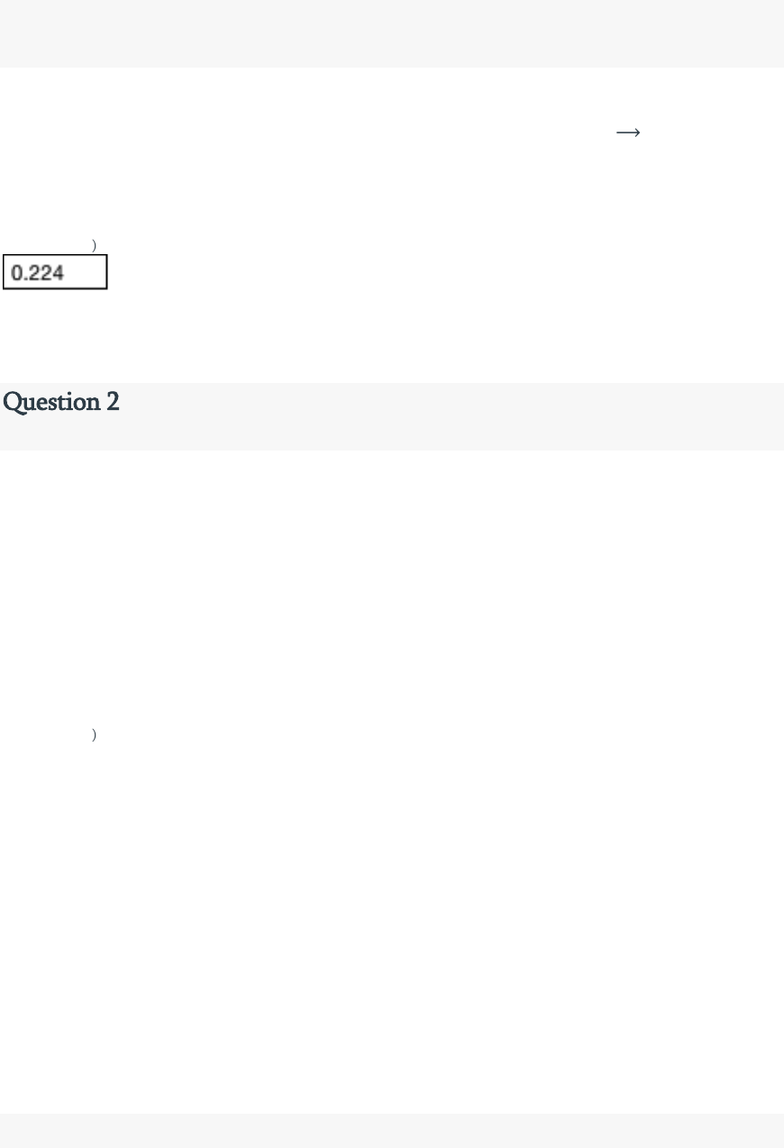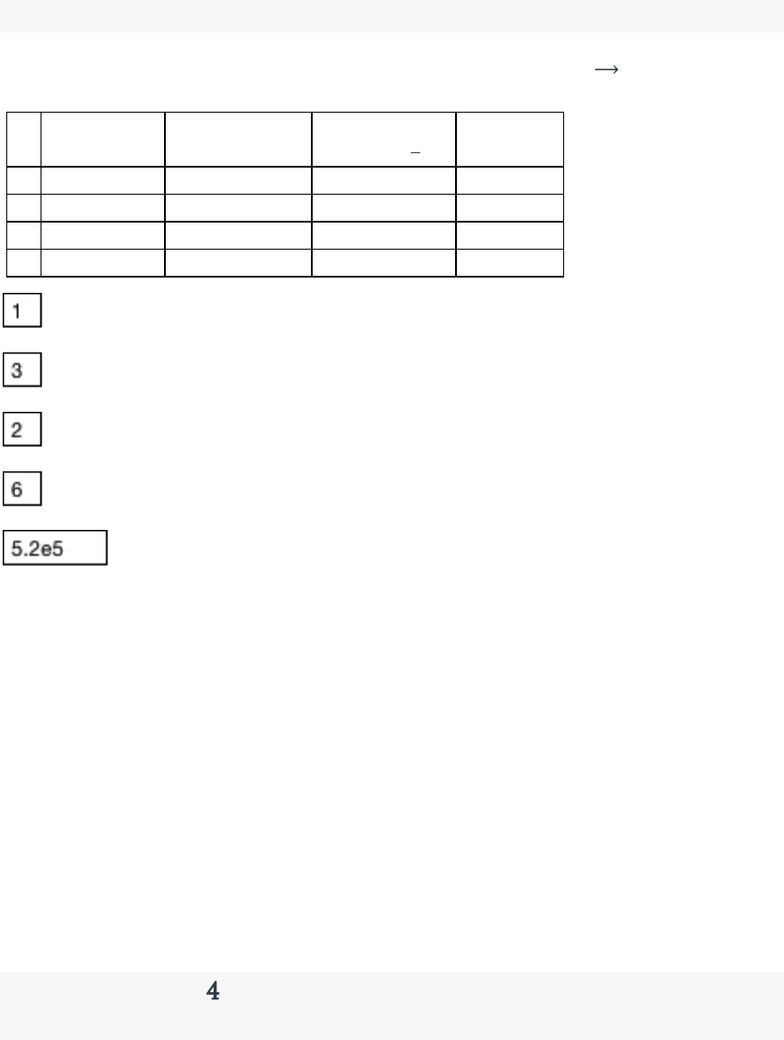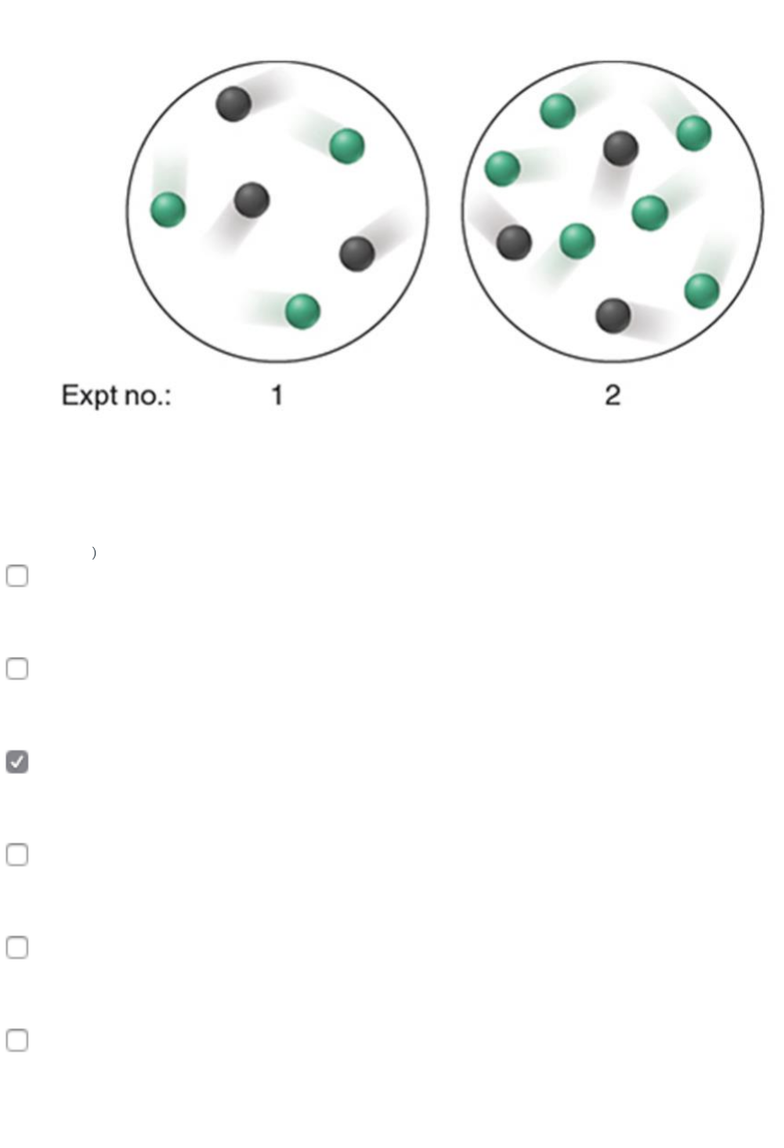# CHM 2046 Chapter Notes - Chapter 16: Rate Equation, Reaction Rate, Joule

30 views8 pages
School
University of Florida
Department
Chemistry
Course
CHM 2046Question 1
1 / 1 pts
Balance the following equation and express the rate in terms of the change in
concentration with time for each substance: NO(g) + O2(g) N2O3 (g)
When N2O3 is forming at 0.112 M/s, at what rate is NO decreasing? Enter a positive
number to 3 decimal places.
(MTS 5/4/2018)
0.224
When the equation is balanced, the reactant to product ratio is 1:2, so if the product
forms at 0.112, the reactant is consumed at twice that rate.
Question 2
1 / 1 pts
Give the individual reaction orders for all substances and the overall reaction order
from the following rate law: rate=k[BrO3−][Br−][H+]2
1 order in bromate ions
1 order in bromide ions
2 order in hydrogen ions
4 overall order of the reaction
(MTS 5/4/2018)
1
1
2
4
Question 3
Unlock document

This preview shows pages 1-3 of the document.
Unlock all 8 pages and 3 million more documents.1 / 1 pts
For the reaction H2SeO3(aq) + 6 I (aq) + 4 H +(aq) Se(s) + 2I3
(aq) + 3 H2O(l) at 0°C, the following data were obtained:
Exp
initial rate (M/s)
initial H2SeO3 (M)
initial I (M)
iniital H+ (M)
1
9.85e-7
0.0025
0.015
0.015
2
7.88e-6
0.0025
0.030
0.015
3
3.94e-6
0.010
0.015
0.015
4
3.15e-5
0.0025
0.030
0.030
order in H2SeO3 (enter a whole number)
order in iodide ion (enter a whole number)
order in hydrogen ion (enter a whole number)
overall order of the reaction (enter a whole number)
what is value of k? Enter a number to 2 sig figs e.g. 1.2e3
1
3
2
6
5.2e5
For 1-3 use the row where all are constant except what you test (Mpers/Mpers =
M/M) and for 5, plug in a full row (initial rate M/s = k (H2SeO3)^x(I)^x(H)^x)
IncorrectQuestion 4
0 / 1 pts
Unlock document

This preview shows pages 1-3 of the document.
Unlock all 8 pages and 3 million more documents.The scenes below show two experiments at a given temperature and volume involving
reactants X (black) and Y (green):
If the rate law for the reaction is rate = k[X]22 what is the initial rate of experiment 2
given that the rate of experiment 1 is 0.50 M/s
(MTS 5/4/2018)
0.50 M/s
0.056 M/s
0.25 M/s
1.0 M/s
0.75 M/s
0.10 M/s